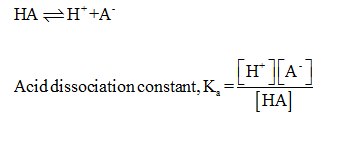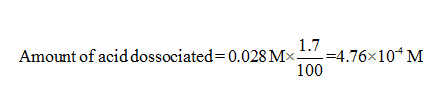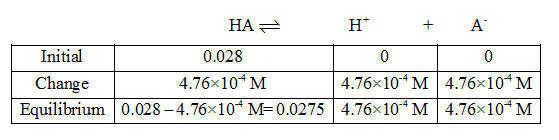# When a 0.028M aqueous solution of a certain acid is prepared, the acid is 1.7% dissociated. Calculate the acid dissociation constant Ka of the acid. Round your answer to 2 significant digits.

Question
1 views

When a 0.028M aqueous solution of a certain acid is prepared, the acid is 1.7% dissociated. Calculate the acid dissociation constant Ka of the acid. Round your answer to 2 significant digits.

check_circle

star
star
star
star
star
1 Rating
Step 1

The acid can be represented as HA. Acid dissociation constant of the reaction is given.Step 2

Initial concentration of the acid is 0.028 M. Here 1.7% of this acid gets dissociated. Amount of acid dissociated can be calculated as given.As the reaction proceeds, it attains equilibrium and hence the concentration can be determined using ICE table....

### Want to see the full answer?

See Solution

#### Want to see this answer and more?

Solutions are written by subject experts who are available 24/7. Questions are typically answered within 1 hour.*

See Solution
*Response times may vary by subject and question.
Tagged in